# HBSE 9th Class Maths Notes Chapter 12 Heron’s Formula

Haryana State Board HBSE 9th Class Maths Notes Chapter 12 Heron’s Formula Notes.

## Haryana Board 9th Class Maths Notes Chapter 12 Heron’s Formula

Introduction
In previous classes, we have studied about various plane figures such as squares, rectangles, triangles, quadrilaterals etc. We have also learnt the formula for finding the areas and the perimeters of these figures like rectangle, square, triangle etc. as given below:
1. Triangle :
(i) $$\frac{1}{2}$$ × Base × Height
$$\frac{1}{2}$$ × BC × AD
(ii) Perimeter = sum of the sides of a triangle
= AB + BC + CA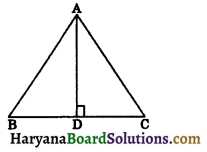2. Right angled triangle:
(i) Area = $$\frac{1}{2}$$ × base × height
= $$\frac{1}{2}$$ × BC × AB
(ii) Perimeter = AB + BC + CA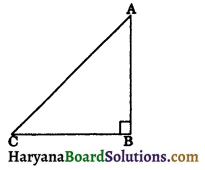3. Equilateral triangle: Let each side of an equilateral triangle be a units.
(i) Area = $$\frac{1}{2}$$ × base × height
= $$\frac{1}{2}$$ × BC × AD
[∵ AD = $$\sqrt{a^2-\frac{a^2}{4}}$$
⇒ AD = $$\sqrt{\frac{3 a^2}{4}}$$
⇒ AD = $$\frac{\sqrt{3} a}{2}$$ ]
= $$\frac{1}{2}$$ × a × $$\frac{\sqrt{3} a}{2}$$
(ii) Perimeter = a + a + a
3a = 3 × side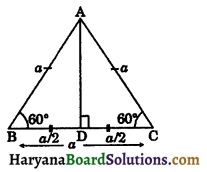4. Parallelogram:
(i) Area = base x height
= AB × DE
(ii) Perimeter = sum of the sides of ||gm
= AB + BC + CD + AD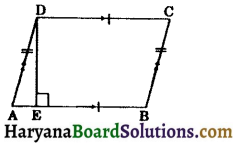5. Square: (i)
Area = side × side = side2
or Area = $$\frac{1}{2}$$ × d2
(where d = diagonal of a square)
(ii) Perimeter = side + side + side + side
= 4 × side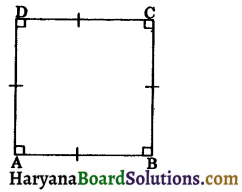6. Rectangle :
(i) Area = base × height
(ii) Perimeter = AB + BC + CD + DA
= L + B + L + B
= 2L + 2B
= 2(L + B)
where L = length of a rectangle
and B = breadth of a rectangle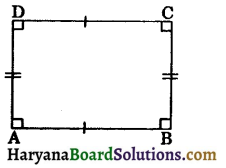7. Rhombus :
(i) Area = base × height
or Area = $$\frac{1}{2}$$ × d1 × d2
where d1 and d2 are the diagonals of a rhombus.
(ii) Perimeter = side + side + side + side
= 4 × side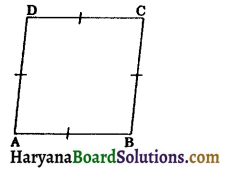8. Trapezium:
(i) Area = $$\frac{1}{2}$$ × (sum of parallel sides) × distance between them
= $$\frac{1}{2}$$ × (AB + DC) × DE
(ii) Perimeter = AB + BC + CD + AD
Unit of measurement for length, breadth, height and perimeter is taken as metre (m) or centimetre (cm), milimetre (mm) etc.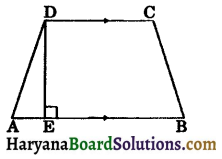Units measurement of Perimeter
1 m = 10 dm, 1 m = 100 cm, 1 m = 1000 mm, 1 km = 10 hm, 1 km = 100 dakm, 1 km = 1000 m Unit of measurement for area of any plane figure is taken as square metre (m2) or square centimetre (cm2) or square millimetre (mm2) etc.
Units measurement of Area
1 km2 = 1 km × 1 km = 10 hm × 10 hm = 100 hm2
1 km2 = 1 km × 1 km = 1000 m × 1000 m = 1000000 m2 = 106 m2
1 hectare = 10000 m2
1 m2 = 1m × 1m = 100 cm × 100 cm = 10000 cm2
1m2 = 1m × 1m = 10 dm × 10 dm = 100 dm2 etc.Key Words
→ Area: The area of a triangle or a polygon is the measure of the surface enclosed by its sides.

→ Perimeter: The perimeter of a plane figure is the length of its boundary.
OR
A perimeter is the sum of lengths of sides of a polygon.

→ Right angled triangle: A triangle with one angle a right angle (90°) is called a right angled triangle.

→ Scalene triangle: If all three sides of a triangle are unequal or different, it is called scalene triangle.

→ Isosceles triangle: If at least two sides of a triangle are equal, it is called isosceles triangle.

→ Equilateral triangle: If all three sides of a triangle are equal, it is called. equilateral triangle.

→ Quadrilateral: A plane figure bounded by four line segments is called a quadrilateral.

→ Parallelogram: A quadrilateral in which opposite sides are parallel and equal is known as a parallelogram.

→ Square: A quadrilateral whose each angle is 90° and all sides are equal to each other is known as a square.

→ Rectangle: A quadrilateral each of whose angles is 90° is called a rectangle.

→ Rhombus: A quadrilateral having all the four sides equal is called a rhombus.

→ Trapezium: A quadrilateral in which one pair of opposite sides are parallel is called a trapezium.

→ Diagonal: A line joining any two vertices of a polygon that are not connected by an edge and which does not go outside the polygon.Basic Concepts
Area of a Triangle by Heron’s Formula: Heron was born in about 10 AD possibly in Alexandria in Egypt. He worked in applied mathematics and wrote three books on mensuration. Book I deals with the area of rectangles, squares, triangles, trapezia, various other specialized quadri- laterals, regular polygons, circles, surfaces of cylinders, cones and spheres etc.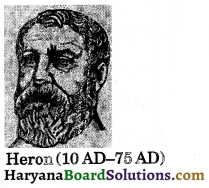He derived the famous formula for finding the area of a triangle in terms of its three sides.
The formula for finding the area of a triangle was given by Heron is stated as below:
Area of a triangle = $$\sqrt{s(s-a)(s-b)(s-c)}$$,
where a, b and c are the sides of a triangle and s = semi-perimeter of the triangle, ie.,
$$\frac{a+b+c}{2}$$
This formula is helpful where it is not possible to find the height of the triangle.Application of Heron’s Formula in Finding Areas of Quadrilaterals: In this section, we have studied the areas of such figures which are the shape of a quadrilaterals. We need to divide the quadrilateral in two triangles and then use the formula 12.25 area of the triangle.